# The tram

The tram is moving with acceleration a = 0.3m/s2. How long will it pass the first meter of the track? How long does it take 10 meters? What is its speed at the end of the 10 meters track?

t1 =  2.582 s
t2 =  8.165 s
v2 =  2.4495 m/s

### Step-by-step explanation:Did you find an error or inaccuracy? Feel free to write us. Thank you!Tips to related online calculators
Do you want to convert velocity (speed) units?
Do you want to convert time units like minutes to seconds?

## Related math problems and questions:

• AccelerationThe car accelerates at a rate of 0.5 m/s2. How long travels 400 meters, and what will be its speed?
• Cyclists and walkersA group of tourists started at 8:00 at speed 4 km/h walk. At half-past ten, another group started on a bike and caught up with a group of tourists at 10:50. What was the average speed of cyclists?
• Car loop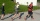The toy car runs at an average speed of 2 m/s. In a minute, it will pass the entire circuit five times. How long is the loop?
• Free fallThe free fall body has gone 10m in the last 0.5s. Find the body speed at the moment of impact.
• Reconstruction of the corridorCalculate how many minutes will be reduced to travel 187 km long railway corridor, where the maximum speed increases from 120 km/h to 160 km/h. Calculate how many minutes will shorten travel time, if we consider that the train must stop at 6 stations, eac
• DriverThe car driver drives at a speed of 100 km/h, saw the obstacle, and began to brake with a slowing of 5 m/s². What is the length of the pathway to stopping the car when the driver has registered the obstacle with a delay of 0.7 s?
• Two cars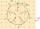Two cars, spaced d1 = 500 m apart, start moving against each other at time t1 = 0, the first car with acceleration a1 = 4 m/s ^ 2 and the second with acceleration a2 = 6 m/s ^ 2. At what time t2 do they meet? What distances s1 and s2 will they move from t
• Two trains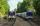Two trains go at each other. First at speed 40 km/h and the second at speed 60 km/h. At what distance will be 30 minutes before they will meet?
• Two airportsTwo airports are 2690 km away. From the first airport, the airplane flies at a speed of 600 km/h, from a second airplane at a speed of 780 km/h. When will they meet if they flew at 10:00? How far from the first airport?
• The swimmerThe swimmer swims at a constant speed of 0.85 m/s relatives to water flow. The current speed in the river is 0.40 m/s, the river width is 90 m. a) What is the resulting speed of the swimmer with respect to the tree on the riverbank when the swimmer's moti
• The positionThe position of a body at any time T is given by the displacement function S=t3-2t2-4t-8. Find its acceleration at each instant time when the velocity is zero.
• Car overtaking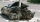A passenger car travels at a speed of 30 m/s, and before it travels freight truck that drives at a constant speed of 10 m/s. Within 30 meters of the truck, the driver of the car finds out that the truck can not overtake. That's why it starts braking with
• Racer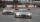The first racer run at a speed 20 m/s and is 750 meters before the finish and ahead 79 meters before second competitor. At what speed must run the second racer to catch first racer at the finish line?
• CarThe driver of a car is to get to 608 km distant city. From atlas found that 162 km will have to pass through the cities at an average speed 48 km/h. The remainder of the journey passes outside the cities at average speed 116 km/h. Calculate how many hours
• CollisionThe two bodies, whose initial distance is 240 m, move evenly against each other consistently. The first body has an initial velocity of 4 m/s and an acceleration of 3 m/s2, the second body has an initial speed of 6 m/s and an acceleration of 2 m/s2. Fin
• Athletic competitionIn a 400 meter athletic competition, a participant covers the distance as given below. find the average speed? first 80 meters 10 m/s next 240 meters 7.5 m/s last 80 meters 10 m/s
• GravitationFrom the top of the 80m high tower, the body is thrown horizontally with an initial speed of 15 m/s. At what time and at what distance from the foot of the tower does the body hit the horizontal surface of the Earth? (use g = 10 ms-2)